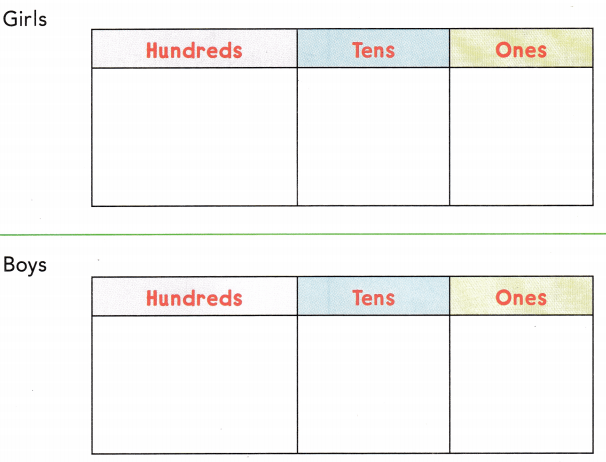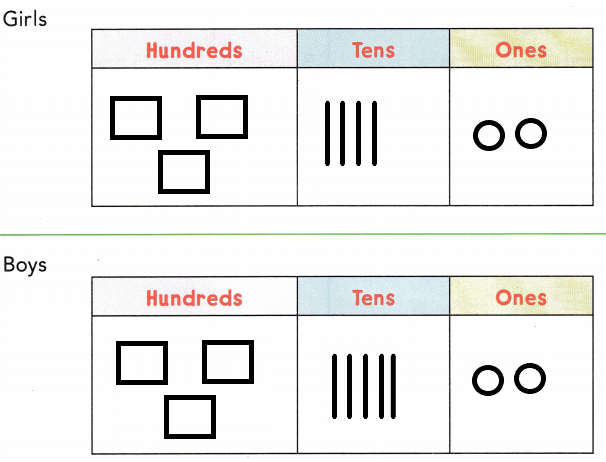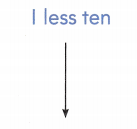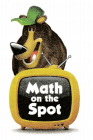# Texas Go Math Grade 2 Lesson 13.2 Answer Key 10 More, 10 Less

Refer to our Texas Go Math Grade 2 Answer Key Pdf to score good marks in the exams. Test yourself by practicing the problems from Texas Go Math Grade 2 Lesson 13.2 Answer Key 10 More, 10 Less.

## Texas Go Math Grade 2 Lesson 13.2 Answer Key 10 More, 10 Less

Explore

Draw quick pictures for the numbers.FOR THE TEACHER
Tell children that there are 342 girls at Center School. Have children draw quick pictures for 342. Then tell them that there are 352 boys at the school. Have them draw quick pictures for 352.

Given that there are 342 girls at center school and 352 boys at the school, so the quick picture for 342 and 352 isMath Talk
Mathematical Processes

Describe how the two numbers are different.

Model and Draw

Use what you know about place value to find 10 more or 10 less than a number.
10 more than 405
4 hundreds 0 tens 5 ones4 hundreds 1 ten 5 ones = ___________

4 hundreds 1 ten 5 ones = 415.

Explanation:
Given the 10 more than 405, so we will add 10 to the given number 405 which is 405 + 10 = 415.

10 less than 405
4 hundreds 0 tens 5 ones3 hundreds 9 tens 5 ones = ___________

3 hundreds 9 tens 5 ones = 395.

Explanation:
Given the 10 less than 405, so we will subtract 10 to the given number 405 which is 405 – 10 = 395.

10 more than 297
2 hundreds 9 tens 7 ones3 hundreds 0 tens 7 ones = ___________

3 hundreds 0 tens 7 ones = 307.

Explanation:
Given the 10 more than 297, so we will add 10 to the given number 297 which is 297 + 10 = 307.

10 less than 297
2 hundreds 9 tens 7 ones2 hundreds 8 tens 7 ones = ___________
2 hundreds 8 tens 7 ones = 287.

Explanation:
Given the 10 less than 297, so we will subtract 10 to the given number 297 which is 297 – 10 = 287.

Share and Show

Write the number

Question 1.
10 more than 287
2 hundreds 9 tens 7 ones = 297.

Explanation:
Given the 10 more than 287, so we will add 10 to the given number 287 which is 287 + 10 = 297.
2 hundreds 8 tens 7 ones2 hundreds 9 tens 7 ones

Question 2.
10 less than 544
5 hundreds 3 tens 4 ones = 534.

Explanation:
Given the 10 less than 544, so we will subtract 10 to the given number 544 which is 544 – 10 = 534.
5 hundreds 4 tens 4 ones5 hundreds 3 tens 4 ones

Question 3.
10 less than 361
3 hundreds 5 tens 1 ones = 351.

Explanation:
Given the 10 less than 361, so we will subtract 10 to the given number 361 which is 361 – 10 = 351.
3 hundreds 5 tens 1 onesQuestion 4.
10 more than 698
7 hundreds 0 tens 8 ones = 708.

Explanation:
Given the 10 more than 698, so we will add 10 to the given number 698 which is 698 + 10 = 708.
6 hundreds 9 tens 8 ones7 hundreds 0 tens 8 ones

Problem Solving

Write the number.

Question 5.
10 more than 914
9 hundreds 2 tens 4 ones = 924.

Explanation:
Given the 10 more than 914, so we will add 10 to the given number 914 which is 914 + 10 = 924.
9 hundreds 1 tens 4 ones9 hundreds 2 tens 4 ones

Question 6.
10 less than 205
1 hundreds 9 tens 5 ones = 195.

Explanation:
Given the 10 less than 205, so we will subtract 10 from the given number 205 which is 205 – 10 = 195.
2 hundreds 0 tens 5 ones1 hundreds 9 tens 5 ones

Question 7.
10 less than 1,044
1 thousand 0 hundreds 3 tens 4 ones = 1,034.

Explanation:
Given the 10 less than 1,044, so we will subtract 10 from the given number 1,044 which is 1,044 – 10 = 1,034.
1 thousand 0 hundreds 4 tens 4 ones1 thousand 0 hundreds 3 tens 4 ones

Question 8.
10 more than 768
7 hundreds 7 tens 8 ones = 778.

Explanation:
Given the 10 more than 768, so we will add 10 to the given number 768 which is 768 + 10 = 778.
7 hundreds 6 tens 8 ones7 hundreds 7 tens 8 ones

Question 9.
10 more than 551
5 hundreds 6 tens 1 ones = 561.

Explanation:
Given the 10 more than 551, so we will add 10 to the given number 551 which is 551 + 10 = 561.
5 hundreds 5 tens 1 ones5 hundreds 6 tens 1 ones

Question 10.
10 less than 1,173
1 thousand 1 hundreds 6 tens 3 ones = 1,163.

Explanation:
Given the 10 less than 1,173, so we will subtract 10 from the given number 1,173 which is 1,173 – 10 = 1,163.
1 thousand 1 hundreds 7 tens 3 ones1 thousand 1 hundreds 6 tens 3 ones

Solve.

Question 11.
H.O.T. Multi-Step Rick has 10 more crayons than Lori. Lori has 36 crayons. Tom has 10 less crayons than Rick. How many crayons does each child have?Rick: _________ crayons
Tom: _________ crayons
Lori: _________ crayons
Rick has 46 crayons,
Tom has 36 crayons,
Lori has 36 crayons.

Explanation:
Given that Lori has 36 crayons and Rick has 10 more crayons, so Rick has 36 + 10 which is 46 crayons. And Tom has 10 fewer crayons than Rick which is 46 – 10 which is 36 crayons. So
Rick has 46 crayons,
Tom has 36 crayons,
Lori has 36 crayons.

Question 12.
H.O.T. Blake wrote the riddles below. Fill in the blanks to make each sentence true.
_________ is 10 less than 1,200 and 10 more than _________. _________ is _________ less than 300.
1,190 is 10 less than 1,200 and 10 more than 1,210. 310 is 10 less than 300.

Explanation:
Here, 1,190 is 10 less than 1,200 and 10 more than 1,210. And 310 is 10 less than 300.

Question 13.
Connect Miss Perez has 135 stickers. Then she gives 10 stickers to students. How many stickers does she have now?
(A) 134
(B) 145
(C) 125
C.

Explanation:
Given that Miss Perez has 135 stickers and then she gives 10 stickers to students. So she has 135 – 10 which is 125.

Question 14.
Mr. Thomas has 124 tickets. He gives some tickets away and has 10 less tickets now. How many tickets does he have now?
(A) 114
(B) 134
(C) 125
A.

Explanation:
Given that Mr. Thomas has 124 tickets and he gives some tickets away and has 10 fewer tickets now. So the number of tickets does he have now is 124 – 10 which is 114.

Question 15.
Analyze Ms. Brooks has a box of 146 bookmarks. Then she buys 10 more bookmarks. How many bookmarks does she have now?
(A) 136
(B) 156
(C) 134
B.

Explanation:
Given that Ms. Brooks has a box of 146 bookmarks and then she bought 10 more bookmarks. So the number of bookmarks does she have now is 146 + 10 which is 156 bookmarks.

Question 16.
TEXAS Test Prep There are 181 pages in Juan’s book. There are 10 more pages in Tina’s book. How many pages are in Tina’s book?
(A) 191
(B) 201
(C) 171
A.

Explanation:
Given that there are 181 pages in Juan’s book and there are 10 more pages in Tina’s book. So the number of pages are in Tina’s book is 181 + 10 which is 191 pages.

### Texas Go Math Grade 2 Lesson 13.2 Homework and Practice Answer Key

Write the number.

Question 1.
10 more than 824
8 hundreds 3 tens 4 ones = 834.

Explanation:
Given the 10 more than 824, so we will add 10 to the given number 824 which is 824 + 10 = 834.
8 hundreds 2 tens 4 ones8 hundreds 3 tens 4 ones

Question 2.
10 less than 307
2 hundreds 9 tens 7 ones = 297.

Explanation:
Given the 10 less than 307, so we will subtract 10 from the given number 307 which is 307 – 10 = 297.
3 hundreds 0 tens 7 ones2 hundreds 9 tens 7 ones

Question 3.
10 less than 1,058
1 thousand 0 hundreds 4 tens 8 ones = 1,048.

Explanation:
Given the 10 less than 1,058, so we will subtract 10 from the given number 1,058 which is 1,058 – 10 = 1,048.
1 thousand 0 hundreds 5 tens 8 ones1 thousand 0 hundreds 4 tens 8 ones

Question 4.
10 more than 678
6 hundreds 8 tens 8 ones = 688.

Explanation:
Given the 10 more than 678, so we will add 10 to the given number 678 which is 678 + 10 = 688.
6 hundreds 7 tens 8 ones6 hundreds 8 tens 8 ones

Question 5.
10 less than 363
3 hundreds 5 tens 3 ones = 353.

Explanation:
Given the 10 less than 363, so we will subtract 10 from the given number 363 which is 363 – 10 = 353.
3 hundreds 6 tens 3 ones3 hundreds 5 tens 3 ones

Question 6.
10 more than 1,137
1 thousand 1 hundreds 4 tens 7 ones = 1,147.

Explanation:
Given the 10 more than 1,137, so we will add 10 to the given number 1,137 which is 1,137 + 10 = 1,147.
1 thousand 1 hundreds 3 tens 7 ones1 thousand 1 hundreds 4 tens 7 ones

Problem Solving

Question 7.
Kayla wrote the riddle below. Fill in the blank to make the sentence true.
___________ is 10 less than 1,148 and 10 more than ____________.
1,138 is 10 less than 1,148 and 10 more than 1,158.

Explanation:
Here, 1,138 is 10 less than 1,148 and 10 more than 1,158.

Question 8.
Multi-Step Joan has 10 more stickers than Todd. Todd has 63 stickers. Alice has 10 less stickers than Joan. How many stickers does each child have?
Joan: ____________
Alice: ____________
Todd: ____________
Joan has 73 stickers,
Alice has 63 stickers,
Todd has 63 stickers.

Explanation:
Given that Todd has 63 stickers, as Joan has 10 more stickers than Todd so Joan has 63 + 10 which is 73 stickers. And Alice has 10 fewer stickers than Joan, so Alice has 73 – 10 which is 63 stickers. So
Joan has 73 stickers,
Alice has 63 stickers,
Todd has 63 stickers.

Lesson Check

Question 9.
Lee has 141 postcards. Rose has 10 more postcards. How many postcards does Rose have?
(A) 161
(B) 151
(C) 131
B.

Explanation:
Given that Lee has 141 postcards and Rose has 10 more postcards, so the number of postcards did Rose had is 141 + 10 which is 151 postcards.

Question 10.
Mr. Brown has a box of 104 paper clips. Then he finds 10 more paper clips in a drawer. How many paper clips does he have now?
(A) 112
(B) 94
(C) 114
C.

Explanation:
Given that Mr. Brown has a box of 104 paper clips and then he finds 10 more paper clips in a drawer, so the number of paper clips does he have now is 104 + 10 which is 114 paper clips.

Question 11.
Harry has 162 trading cards. He gives 10 trading cards to his brother. How many trading cards does Harry have now?
(A) 152
(B) 142
(C) 172
A.

Explanation:
Given that Harry has 162 trading cards and he gives 10 trading cards to his brother, so the number of trading cards does Harry have now is 162 – 10 which is 152 trading cards.

Question 12.
Mrs. Ryan has 165 gold stickers. She gives 10 gold stickers to students. How many gold stickers does she have left?
(A) 164
(B) 175
(C) 155